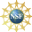# 8. Properties of Curves

## 2. Torsion

The torsion of a curve, denoted by the Greek letter tau, $$\tau$$, is a measure of how quickly the instantaneous plane of a curve is twisting. Unlike the curvature $$\kappa$$ (which is always positive), the torsion can be positive or negative to measure the handedness of the curve. For example, the two helices at the right are traversed from bottom to top. However, when looked at from the top (positive $$z$$-axis), the first is traversed clockwise (left handed) while the second is traversed counterclockwise (right handed). If you wrap the fingers of your right hand around the helix with your fingers in the direction the curve is traversed, your thumb will point down for the first helix and up for the second helix. This handedness is reflected in the torsion which is negative for the first helix and positive for the second helix.

The unit binormal vector, $$\hat{B}$$, is the normal vector to the instantaneous plane of the curve. So the rate of change of the orientation of the plane is measured by $$\dfrac{d\hat{B}}{ds}$$. However, if we define the torsion as its magnitude $$\left|\dfrac{d\hat{B}}{ds}\right|$$ (like we did for the curvature), then it would always be positive and we want its sign to determine the handedness of the twist. So we need a slightly different definition.

The torsion, $$\tau$$, of a parametric curve is $\tau=-\,\dfrac{d\hat{B}}{ds}\cdot\hat{N}$ where $$\hat{N}$$ is the unit normal vector, $$\hat{B}$$ is the unit binormal vector and $$s$$ is the arc length parameter on the curve.

1) We take the derivative with respect to arc length $$s$$ instead of the parameter $$t$$ because we want the torsion to be geometrical and not depend on the speed we traverse the curve.

2) The minus sign is needed to ensure that the torsion of a right handed helix is positive and that of a left handed helix is negative. The helicity of a curve is defined to be the sign of the torsion.

3) We dot with $$\hat{N}$$ because, as we will see on the page about Frenet Frames, $$\dfrac{d\hat{B}}{ds}=-\tau\hat{N}$$, and this is the best way to isolate the coefficient $$-\tau$$. In fact, if we take the length of this formula, we get $|\tau|=\left|\dfrac{d\hat{B}}{ds}\right|$ which could be used as the definition of the torsion, except for its sign which is the helicity.

It is often easier to calculate torsion using the following formula:

The torsion can be calculated from: $\tau=\dfrac{\vec{v}\times\vec{a}\cdot\vec j}{|\vec{v}\times\vec{a}|^2}$

Note: The $$\vec j$$ in this formula is the jerk, not the unit vector $$\hat j$$ in the $$y$$ direction!

Find the torsion $$\tau$$ of the right handed helix $$\vec{r}=(4\cos\theta,4\sin\theta,3\theta)$$. See the second figure at the top. Is the torsion positive or negative?

On a previous page, in the example to find $$\hat{T}$$, $$\hat{N}$$ and $$\hat{B}$$ for this right handed helix, we found: $\vec{v}=\langle -4\sin\theta,4\cos\theta,3\rangle \qquad \vec{a}=\langle -4\cos\theta,-4\sin\theta,0\rangle$ $\vec{v}\times\vec{a}=\langle 12\sin\theta,-12\cos\theta,16\rangle \qquad |\vec{v}\times\vec{a}|=20$ So: $\vec{j}=\langle 4\sin\theta,-4\cos\theta,0\rangle \qquad \vec{v}\times\vec{a}\cdot\vec{j}=48\sin^2\theta+48\cos^2\theta=48$ and: $\tau=\dfrac{\vec{v}\times\vec{a}\cdot\vec{j}}{|\vec{v}\times\vec{a}|^2} =\dfrac{48}{20^2}=\dfrac{3}{25}$ We see the torsion is positive.

Find the torsion $$\tau$$ of the left handed helix $$\vec{r}=\langle 4\cos\theta,-4\sin\theta,3\theta\rangle$$. See the first figure at the top. Is the torsion positive or negative?

$$\tau=\dfrac{-3}{25}$$   We see the torsion is negative.

We compute: $\vec{v}=\langle -4\sin\theta,-4\cos\theta,3\rangle \qquad \vec{a}=\langle -4\cos\theta,4\sin\theta,0\rangle$ \begin{aligned} \vec{v}\times\vec{a} &=\langle -12\sin\theta,-12\cos\theta,-16\sin^2\theta-16\cos^2\theta\rangle \\ &=\langle -12\sin\theta,-12\cos\theta,-16\rangle \end{aligned} $|\vec{v}\times\vec{a}| =\sqrt{144\sin^2\theta+144\cos^2\theta+256}=20$ $\vec{j}=\langle 4\sin\theta,4\cos\theta,0\rangle$ $\vec{v}\times\vec{a}\cdot\vec{j} =-48\sin^2\theta-48\cos^2\theta=-48$ So $\tau=\dfrac{\vec{v}\times\vec{a}\cdot\vec{j}}{|\vec{v}\times\vec{a}|^2} =\dfrac{-48}{20^2}=\dfrac{-3}{25}$ We see the torsion is negative.

From this example and exercise, notice that the torsion of the right handed helix is positive while that of the left handed helix is negative.

Find the torsion $$\tau$$ of the twisted cubic $$\vec{r}=(t,t^2,\dfrac{2}{3}t^{3})$$. Is it right handed or left handed? See the example on a previous page.

$$\tau=\dfrac{2}{(1+2t^2)^2}$$

On a previous page, in the example to find $$\hat{T}$$, $$\hat{N}$$ and $$\hat{B}$$ for this twisted cubic, we found: $\vec{v}=\langle 1,2t,2t^2\rangle \qquad \vec{a}=\langle 0,2,4t\rangle$ $\vec{v}\times\vec{a}=\langle 4t^2,-4t,2\rangle \qquad |\vec{v}\times\vec{a}|=2(1+2t^2)$ Then: $\vec{j}=\langle 0,0,4\rangle \qquad \vec{v}\times\vec{a}\cdot\vec{j}=8$ and so: $\tau=\dfrac{\vec{v}\times\vec{a}\cdot\vec{j}}{|\vec{v}\times\vec{a}|^2} =\dfrac{8}{4(1+2t^2)^2}=\dfrac{2}{(1+2t^2)^2}$ Since the torsion is positive the curve is right handed.

Find the torsion $$\tau$$ of the circle $$\vec{r}=\langle R\cos\theta,R\sin\theta,0\rangle$$. See the example on a previous page. Why should you have expected this answer?

The torsion is $$\tau=0$$, which should be expected because the plane of a circle does not change

On a previous page, in the example to find $$\hat{T}$$, $$\hat{N}$$ and $$\hat{B}$$ for this circle, we found: $\vec{v}=\langle -R\sin\theta,R\cos\theta,0\rangle \qquad \vec{a}=\langle -R\cos\theta,-R\sin\theta,0\rangle$ $\vec{v}\times\vec{a}=\langle 0,0,R^2\rangle \qquad |\vec{v}\times\vec{a}|=R^2$ Using these, $\vec{j}=\langle R \sin\theta,-R\cos\theta, 0\rangle \qquad \vec{v}\times\vec{a}\cdot\vec{j}=0$ So $\tau=\dfrac{\vec{v}\times\vec{a}\cdot\vec{j}}{|\vec{v}\times\vec{a}|^2}=0$ Thus, the torsion is zero, which should be expected because the plane of a circle does not change

Supported in part by NSF Grant #1123255